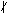# divides

We say one integer divides another if it does so evenly, that is with a remainder of zero (we sometimes say, "with no remainder," but that is not technically correct).  More formally, mathematicians write:

If a and b are integers (with a not zero), we say a divides b if there is an integer c such that b = ac.

We use this concept enough that it has its own symbols:

 a | b means a divides b. ab means a does not divide b.

The integers that divide a are called the divisors of a.

You might want try your hand at proving the following basic properties which hold for all integers a, b. c and d:

1. a | 0, 1 | a, a | a.
2. a | 1 if and only if a=+/-1.
3. If a | b and c | d, then ac | bd.
4. If a | b and b | c, then a | c.
5. a | b and b | a if and only if a=+/-b.
6. If a | b and b is not zero, then |a| < |b|.
7. If a | b and a | c, then a | (bx+cy) for all integers x and y.

Finally, suppose p is a prime and k is a positive integer.  A notation that is quickly gaining acceptance is to write pk || a to indicate that pk divides a, but pk+1 does not.